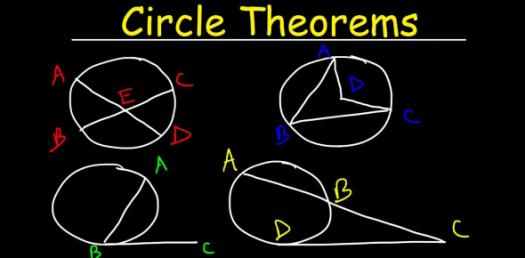# Can You Pass This Circle Theorems Test? Math Quiz

20 Questions | Total Attempts: 175SettingsWorking out angles in circles

Related Topics
• 1.
A = [Blank]o
• 2.
B = [Blank]o
• 3.
C = [Blank]o
• 4.
D = [Blank]o
• 5.
A = [Blank]o
• 6.
B = [Blank]oc = [Blank]o
• 7.
D = [Blank]oe = [Blank]of = [Blank]o
• 8.
G = [Blank]oh = [Blank]oi = [Blank]o
• 9.
A = [Blank]ob = [Blank]oc = [Blank]o
• 10.
X = [Blank]oy = [Blank]oz = [Blank]o
• 11.
X = [Blank]oy = [Blank]o
• 12.
X = [Blank]oy = [Blank]oz = [Blank]o
• 13.
X = [Blank]oy = [Blank]o
• 14.
X = [Blank]o
• 15.
Angle DBC = [Blank]oAngle CDA = [Blank]oAngle DCA = [Blank]o
• 16.